The mathematical pendulum

We follow Gerd Baumann's approach in Mathematica to determine the period of the mathematical pendulum.

First, we write down the kinetic and potential energies: the kinetic energy is expressed in terms of the angular velocity and the moment of inertia for a point mass m suspended at distance l .

We call the angular velocity d/dt phi=omega

> restart;

> Tkin:=1/2*m*l^2*omega^2;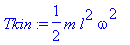The potential energy follows from the height of the pendulum bob (point mass m ). We choose the potential energy to be zero if the mass is at the bottom.

> Upot:=m*g*l*(1-cos(phi));For plotting we have to pick some parameter values:

> l:=1;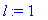> g:=10;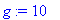> m:=1;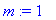> plots[contourplot](Tkin+Upot,phi=-3/2*Pi..3/2*Pi,omega=-10..10,contours=[1,5,9,13,17,21,25,29],axes=boxed,filled=true, coloring=[red,green]);This phase space diagram is obviously periodic with period 2 Pi. For small angular velocities one has a system approximated by a harmonic oscillator, while for larger velocites (or energies, i.e., higher contours) one has solutions that simply revolve (winding the pendulum; in the periodic phase space diagrams one leaves e.g., at the right to re-enter at the same angular velocity at the left. The motion is left to right in the top half (for the open curves), and right to the left in the bottom half (look at the sign of the angular velocity).

The regularity of the diagram is very striking. The units of energy (chosen for the contour lines) are in MKSA, if l , g , m are given in MKSA.

There is a critical energy where the separation occurs between oscillating solutions (closed contours, in the somewhat abstract phase space one obtains elliptic or deformed elliptic 'trajectories'; translate those into oscillations using your imagination, by watching the sign of omega, passage through phi=0, i.e., the bottom position of the pendulum, etc.)

The locations phi=+Pi, omega=0, or phi=-Pi, omega=0 correspond to the unstable equilibrium (pendulum at the top at rest). One can show that the phase-space 'trajectory' become a cosine curves of phi/2 [Marion-Thornton, eq. 4.31].

For winding solutions we observe how their angular velocity is modulated as the go through the (periodic) potential well. For sufficiently high energies (not shown) the phase space 'trajectories' approach straigh lines, as the pendulum bob's kinetic energy is so high that the variation in the potential energy (by being moved up and down in the graviational field) becomes negligible.

Time-dependent perturbations of the pendulum by an external force can lead to a loss of regularity in the phase space diagram (CHAOS). The modulation can come from a simple coupling to a second pendulum, which leads to a time-varying support point (science museum demonstrations + classroom demo!).

The determination of the phase-space 'trajectories' was easy. The actual integration of phi(t) is not!

The total energy can be expressed through the potential energy at the turning point (pendulum bob at rest), i.e., through the initial angle phi0.

One introduces the energy parameter (initial phase angle phi0) k=sin(phi0/2);

To recover the general expression for the potential energy we unassign the parameters for length, gravitational acceleration, and mass:

> l:='l': g:='g': m:='m': Upot;> Upot:=algsubs(cos(phi)=1-2*sin(phi/2)^2,Upot);> Etot:=subs(phi=phi0,Upot);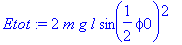The total energy for a given trajectory thus depends only on k =sin(phi/2). Therefore, the period is calculated as a function of k .

To calculate the period for the oscillatory solutions, one uses the energy expression which depends on phi and omega=diff(phi,t), isolates omega.

> Tkin+Upot=Etot;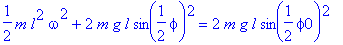> solve(%,omega);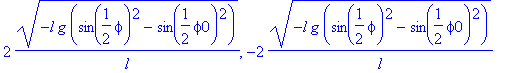> DE:=diff(phi(t),t)=subs(phi=phi(t),%);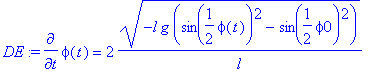> sol:=dsolve({DE,phi(0)=phi0},phi(t));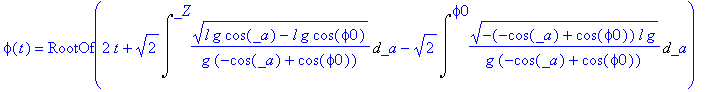Maple 5.0 comes up with a formal solution for phi(t). It hints at the possibility of finding the solution through elliptic integrals. In Maple 6.0 no answer is returned after the program tries to find a solution for a while.

For the determination of the period T one can proceed differently: the differential equation DE can be separated so that an expression for dt is obtained:

> subs(diff(phi(t),t)=dphi/dt,DE);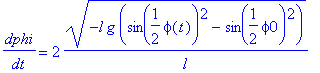> solve(%,dt);If we integrate the right-hand side over phi from 0 to phi0, the left-hand side will give us a quarter of the period T (for the full period phi would have to continue through 0 to -phi0 and back to phi0.

The integral to be carried out falls into a category known as elliptic.

> assume(k>0);

A required integral can be expressed as an elliptic function:

> Int(1/sqrt((1-z^2)*(1-k^2*z^2)),z=0..1);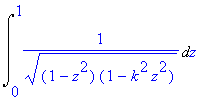> value(%);> omega:=sqrt(g/l);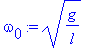To help Maple we restrict the inital phase to be positive, and less than Pi.

> assume(phi0>0,phi0<Pi);

> T:=2/omega*Int(1/sqrt(sin(phi0/2)^2-sin(phi/2)^2),phi=0..phi0);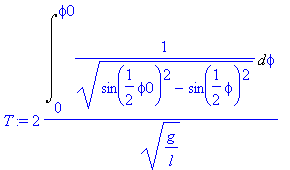> T:=value(T);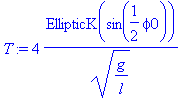For plotting purposes let us simplify matters by chosing the pendulum length and the gravitational acceleration in MKSA units:

> g:=10; l:=10;> T;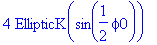We now explore this answer as a function of the initial angle phi0:

> plot(T,phi0=0..Pi);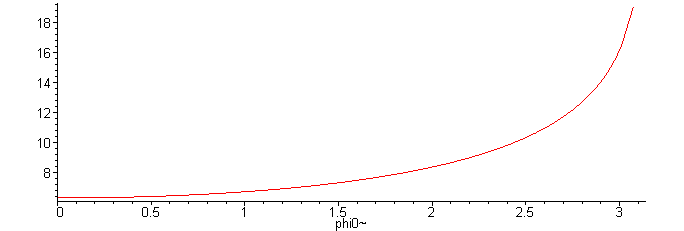In the limit of the intial phase going to Pi (unstable equilibrium at the top) we obtain an infinite period:

> limit(T,phi0=Pi,left);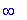What do we get for small initial phase angles (we should recover isochronicity in the small-amplitude limit)

> plot(T,phi0=0..Pi/16);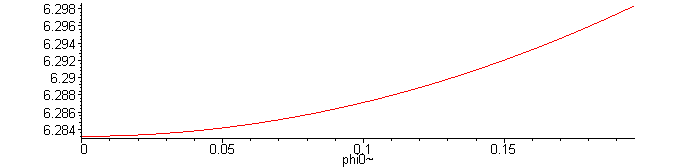> taylor(T,phi0=0,5);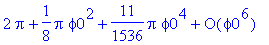> T1:=algsubs(sin(phi0/2)=k,T);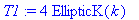> series(T1,k=0,8);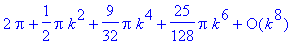> T1t:=convert(%,polynom);This agrees with eq. 2.25 in Baumann (or analytical mechanics texts).

> plot({Re(T1),T1t},k=0..1);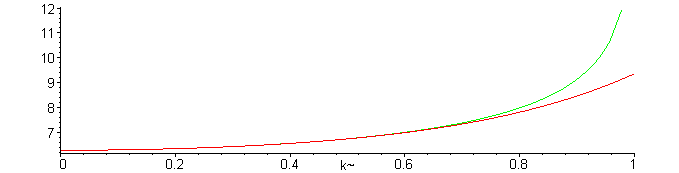An alternative would be to introduce the variable k directly in the integrand

> T2:=2/omega*int(1/sqrt(k^2-sin(phi/2)^2),phi=0..2*arcsin(k));> simplify(T2);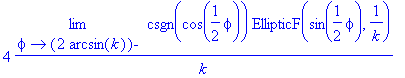This does not work in Maple5!

> simplify(T2,symbolic);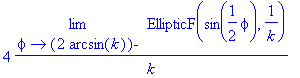Maple is reluctant to carry out the cancellation of function and its inverse. In Maple6 T2 is directly calculated as 4*EllipticK(k).

A further exploration should look into the calculation of trajectories phi( t )!

Before we proceed with this step we construct the phase diagram (a plot of the time derivative of the dynamic variable [the phase angle phi] against the dynamic variable itself. For this we need the energy expression (we will look at contour lines of equal height, i.e. lines of constant energy, as the mechanical energy is conserved for a frictionless mathematical pendulum).

> l:='l': g:='g': m:='m':

> phi:='phi';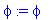> DE;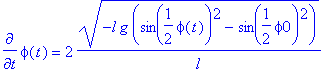Let us make use of Gerd Baumann's analytic work:

> omega:=sqrt(g/l);> Etot;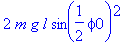> E_s:=Etot/(m*l^2*omega^2);The scaled energy parameter allows to distinguish easily the different types of motion: for E_s<2 we have oscillations, for E_s>2 rotations, and E_s=2 corresponds to the asymptotic solution where the pendulum bob remains in the unstable equilibrium point.

We express the scaled energy in terms of the parameter k =sin(phi0/2):

> k0:=7/8;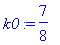> E_s:=2*k0^2;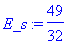> phi:='phi':

We define the differential equation such that positive and negative angular velocities are allowed.

> DE:=(diff(phi(t),t))^2=omega^2*(2*(cos(phi(t))-1+E_s));Oscillatory solutions to the equation of motion (E_s<2) are given by:

> theta:=(k,t)->2*arcsin(k*JacobiSN(omega*t,k));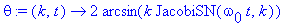> g:=10; l:=1;> subs(phi(t)=theta(k0,t),DE);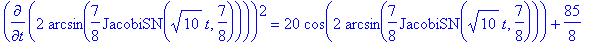> simplify(%);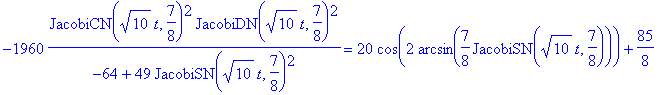> evalf(subs(t=1,%));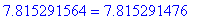Without further work Maple cannot figure out that the expression written for theta( t ) solves the differential equation. Nevertheless, we can verify that within numerical accuracy the differential equation is satisfied.

> plot([theta(0.5,t),theta(0.8,t),theta(0.9,t),theta(0.999,t)],t=0..2*Pi,color=[red,blue,green,black],view=[0..2*Pi,-Pi..Pi]);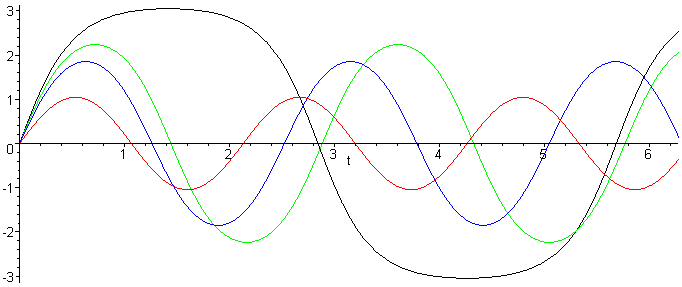One observes that for moderate energy values the solution still looks like a sine-curve, although the dependence of the period on the energy is apparent already. Only for values of k close to unity do we see a qualtiative change in the behaviour of the solution, i.e., a dramatic slowdown of the pendulum bob in the vicinity of the turning point.

We can follow this up with an animation. To display the pendulum's motion we need to calculate the x and y coordinates. We choose a length of l =1 and define the coordinates such that the height is zero at the suspension point of the pendulum.

> x:=(k,t)->evalf(sin(theta(k,t))): y:=(k,t)->-evalf(cos(theta(k,t))):

> x(0.99,1),y(0.99,1);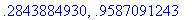We draw lines from the origin to the pendulum bob:

> PL:=(k,t)->plot([[0,0],[x(k,t),y(k,t)]],style=line,color=magenta,title=cat(cat("k= ",convert(evalf(k,3),string)),"time= ",convert(evalf(t,3),string))):

> PLs:=seq(PL(0.995,it/10),it=1..200):

> with(plots):

> display(PLs,insequence=true,axes=boxed,scaling=constrained);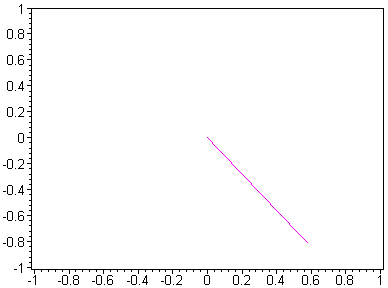The slowdown of the pendulum bob as the top position is approached is very obvious. Thus the lack of isochronicity, or the dependence of the period on the maximum amplitude (energy deposited into the pendulum) is made very obvious.

In Maple 6 the title for each frame is actually displayed in the animation. However, we found two errors for Maple 6.0 in this worksheet: 1) the contourplot is wrong; 2) the numerical evaluation of the JacobiSN functions is in error. We can only hope that a service pack will remedy these problems.

While the demonstration of Maple's handling of elliptic integrals has been impressive, we wish to demonstrate also a general approach that can be applied to any differential equation, namely the numerical solution.

> DE:=k->(diff(phi(t),t))^2=omega^2*(2*(cos(phi(t))-1+2*k^2));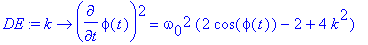> omega:=1;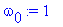> IC:=phi(0)=0;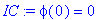> sol:=dsolve({DE(0.999),IC},phi(t),numeric);

`Error, (in DEtools/convertsys) unable to convert to an explicit first-order system`

Bad news! We need to go back to the original Newton law. Maple 6 is, however, improved in this area.

> omega:='omega': # it is not good to mix omega(t), and omega, which makes omega a table.

> DE:=diff(phi(t),t)=omega(t),diff(omega(t),t)=-(g/l)*sin(phi(t));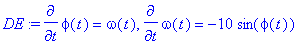The initial condition is chosen to be the turning point, where all energy is in the potential.

> phifun:=k->2*arcsin(k);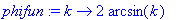> phi0:=phifun(0.999);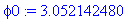> IC:=omega(0)=0,phi(0)=phi0;> sol:=dsolve({DE,IC},[omega(t),phi(t)],numeric,output=listprocedure);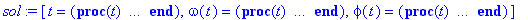> theta:=subs(sol,phi(t));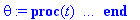In the plot command we need to put quotes around theta(t), as the expression can only be evaluated for numerical values of t.

> plot('theta(t)',t=0..2*Pi);> Omega:=subs(sol,omega(t));> plot('Omega(t)',t=0..2*Pi);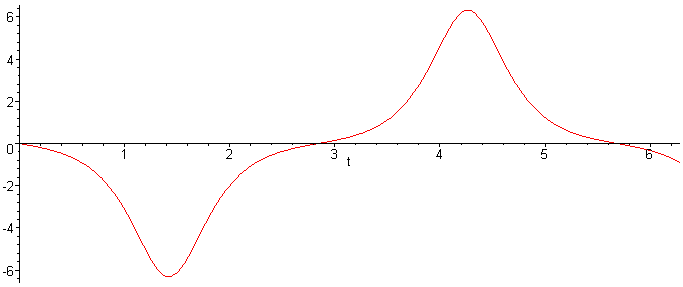It is evident that there are extended time segments when the pendulum bob moves with low velocities. A useful exercise would be to reduce the parameter k , and to observe when the relationship between angular velocity and angle returns to that of a harmonic oscillator (sine/cosine curves).

We can also look at the solutions where the pendulum bob rotates.

> theta:=(t,k)->2*JacobiAM(sqrt(g/l)*t,k);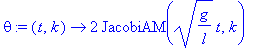> g:=10; l:=1;> plot(Re(theta(t,0.6*I)),t=0..4);> evalf(theta(1,5.5*I));The imaginary values of k come from the following analytic continuation of the energy-initial angle relation:

> E_s:=2.1;> solve(1-E_s=cos(phi1),phi1);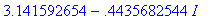> k:=evalf(sin(%));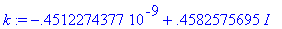Exercise: Adapt the animation to display the motion in the case where the pendulum bob has sufficient energy to rotate over the top position.

>